# Time Unit Conversion Worksheet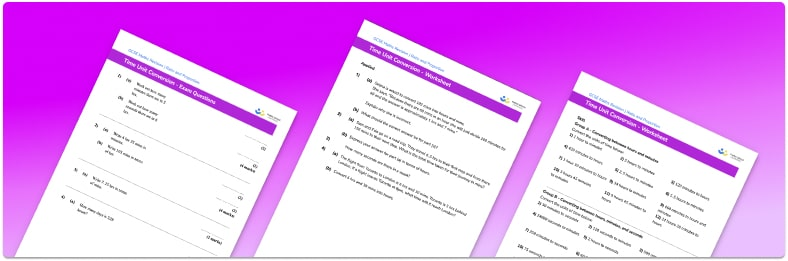• Section 1 of the time unit conversion worksheet contains 27 skills-based time unit conversion questions, in 3 groups to support differentiation
• Section 2 of the time worksheet contains 3 applied time unit conversion questions with a mix of time word problems and deeper problem solving questions
• Section 3 contains 4 foundation and higher level GCSE exam style time unit conversion questions
• An answer key and a mark scheme for all time unit conversion questions are provided
• Questions follow variation theory with plenty of opportunities for students to work independently at their own level
• All questions created by fully qualified expert secondary maths teachers
• Suitable for GCSE maths revision for AQA, OCR and Edexcel exam boards

• This field is for validation purposes and should be left unchanged.

You can unsubscribe at any time (each email we send will contain an easy way to unsubscribe). To find out more about how we use your data, see our privacy policy.

### Time unit conversion at a glance

Time unit conversion involves converting between different units of time. It is important to remember that, unlike the metric system, time does not work in powers of 10

The time conversion rates are:

• 60  seconds = 1  minute
• 60 minutes = 1  hour
• 24 hours = 1 day
• 7 days = 1 week
• 365 days = 1 year

Converting to smaller or larger units can make a measurement of time easier to understand. For example, 14400 seconds is much easier to understand when it is converted to 4 hours.

Mistakes can often be made when converting hours to minutes and converting minutes to seconds or vice versa.

To convert hours to minutes we must multiply by 60 , for example 1.5  hours = 1.5 \times 60 = 90  minutes. To convert hours to minutes, we must divide by 60 , for example 75 minutes = 1.25  hours  = 1 hour 15  minutes. Notice here that 0.25 hours is 15 minutes (a quarter of an hour) not 25  minutes as 60 \times 0.25 = 15

In order to solve problems involving time unit conversion, students should be confident in telling time and the 24 hour clock.

Looking forward, students can then progress to additional conversion worksheets and other ratio and proportion worksheets, for example a ratio worksheet or a simplifying and equivalent ratios worksheet.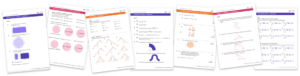For more teaching and learning support on Ratio and Proportion our GCSE maths lessons provide step by step support for all GCSE maths concepts.

## Related worksheets

Unit Conversion Worksheet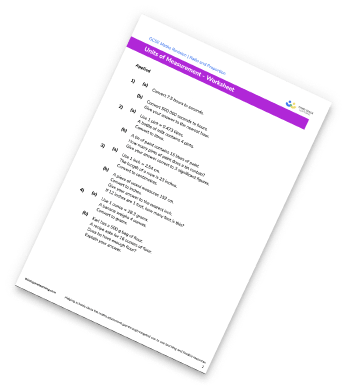Converting Units Of Area And Volume Worksheet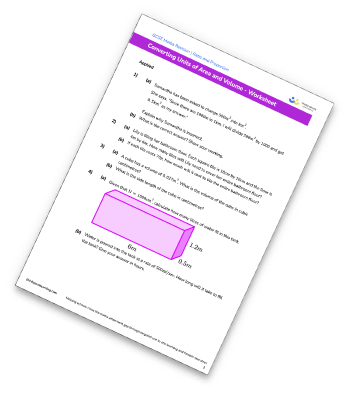Converting Units Of Measure Worksheet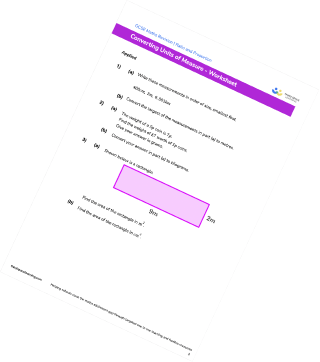Metric Conversion Worksheet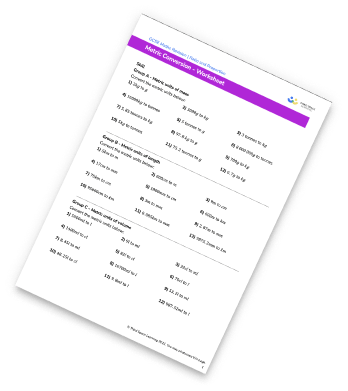## Do you have KS4 students who need more focused attention to succeed at GCSE?There will be students in your class who require individual attention to help them succeed in their maths GCSEs. In a class of 30, it’s not always easy to provide.

Help your students feel confident with exam-style questions and the strategies they’ll need to answer them correctly with our dedicated GCSE maths revision programme.

Lessons are selected to provide support where each student needs it most, and specially-trained GCSE maths tutors adapt the pitch and pace of each lesson. This ensures a personalised revision programme that raises grades and boosts confidence.

Find out more